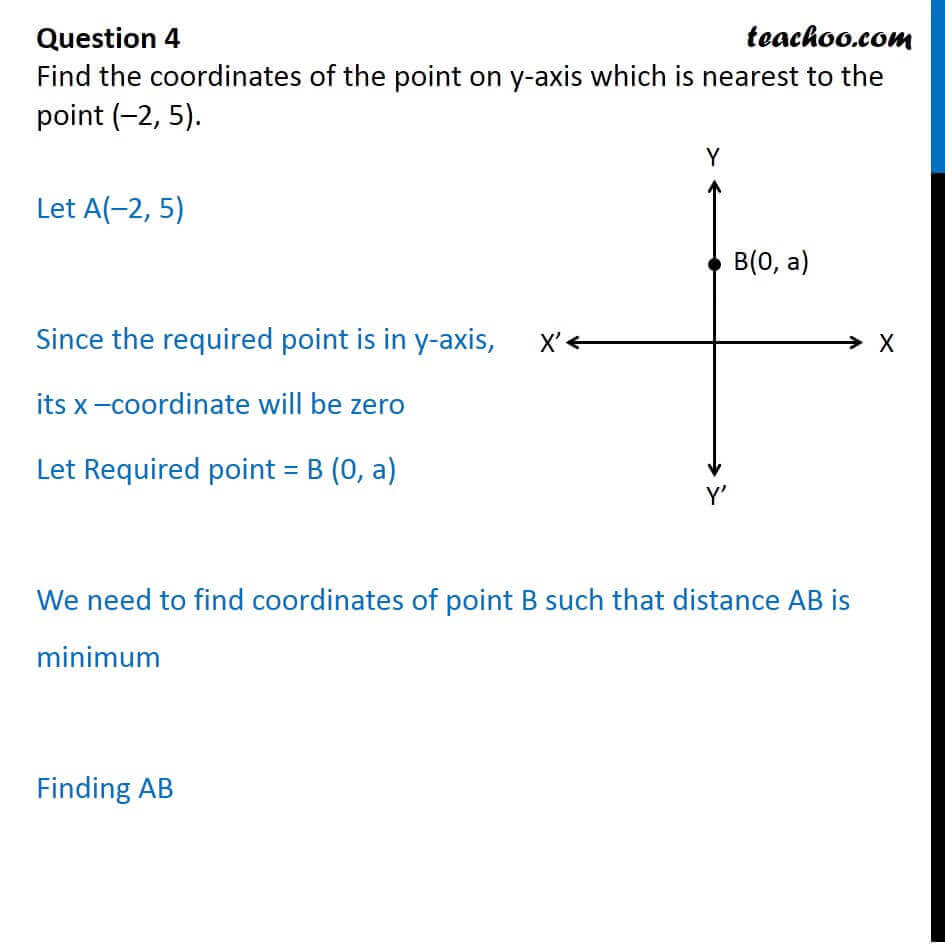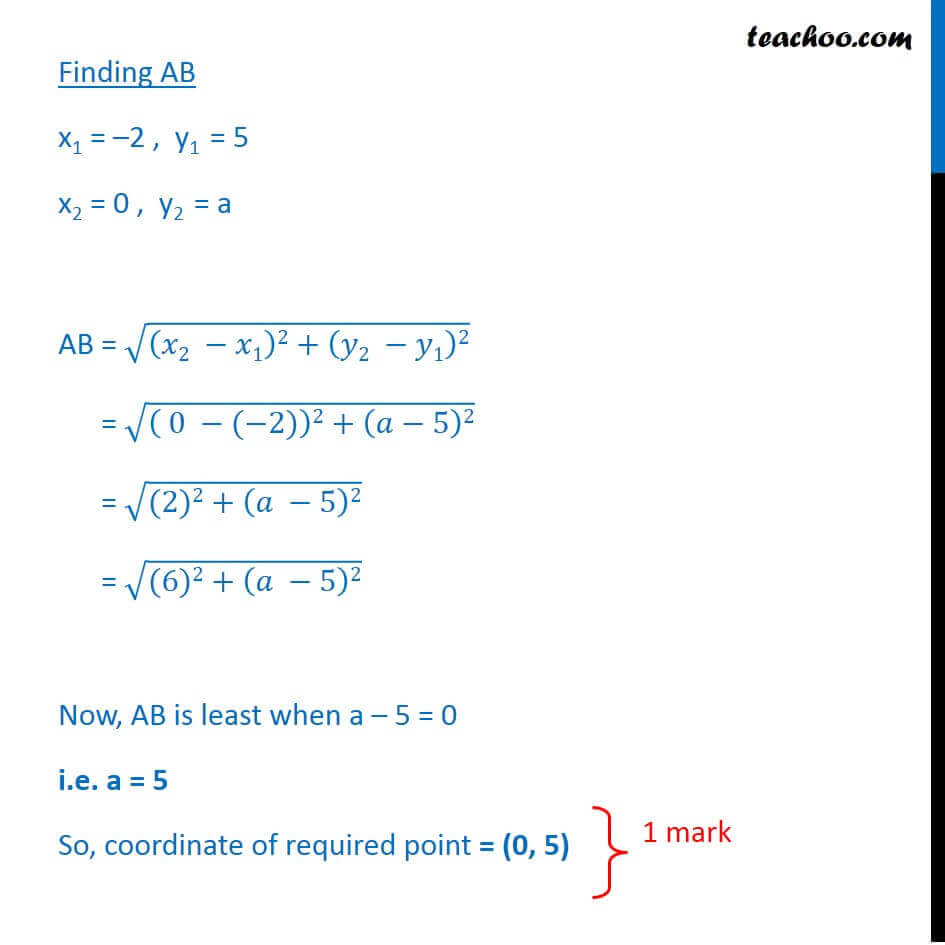CBSE Class 10 Sample Paper for 2018 Boards

Class 10
Solutions of Sample Papers for Class 10 Boards

### Find the coordinates of the point on y-axis which is nearest to the point (–2, 5).

This is a question of CBSE Sample Paper - Class 10 - 2017/18.Learn in your speed, with individual attention - Teachoo Maths 1-on-1 Class

### Transcript

Question 4 Find the coordinates of the point on y-axis which is nearest to the point ( 2, 5). Let A( 2, 5) Since the required point is in y-axis, its x coordinate will be zero Let Required point = B (0, a) We need to find coordinates of point B such that distance AB is minimum Finding AB Finding AB x1 = 2 , y1 = 5 x2 = 0 , y2 = a AB = (( 2 1)2+( 2 1)2) = (( 0 ( 2))2+( 5)2) = ((2)2+( 5)2) = ((6)2+( 5)2) Now, AB is least when a 5 = 0 i.e. a = 5 So, coordinate of required point = (0, 5)